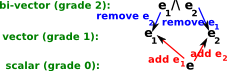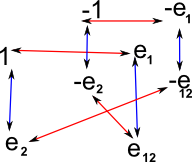# Maths - 2D Clifford / Geometric Algebra

## Description

On the previous pages we showed that from a two dimensional vectors we generate an algebra with 4 elements (e, e1, e2and e1^e2) .

e scalar
e1, e2 vector
e1^e2 bivector (in this case a scalar value)

We can define a general 'number' in this algebra as a linear sum of these basis:

a + b e1 + c e2+ d e1^e2

We will call this 'number' a multivector, and for 2D vectors is is defined by the 4 scalar values here denoted by a,b,c and d.

 In order to understand the properties of this algebra we need to look at the arithmetic rules here.Such an algebra is the smallest, non-trivial clifford algebra so this may be a good chance to experiment with what we can change.

For instance, we can try changing the following aspects of the algebra:

• Do the basis vectors square to +ve, -ve or zero?
• Do we choose e1^e2 or e2^e1 as the basis bivector?
• Do the components, not on the leading diagonal, anti commute?

These options are discussed on this page.

## Cayley Table and Graph

If the basis vectors square to +ve then the Cayley table and graph are as follows:

Cayley Table
Cayley Graph
 a*b b.e b.e1 b.e2 b.e12 a.e 1 e1 e2 e12 a.e1 e1 1 e12 e2 a.e2 e2 -e12 1 -e1 a.e12 e12 -e2 e1 -1or as a hypercube:There are 2 generators since e1 and e2 are the generators.

## Even subalgebra

If we take the even subalgebra (scalar and bivector) then the Cayley table and graph are as follows:

Cayley Table
Cayley Graph
 a*b b.e b.e12 a.e 1 e12 a.e12 e12 -1This is isomorphic to the algebra of complex numbers, it has one generator: e12

## Comparison of 2D multivector with Quaternion

Clifford algebras based on 2D vectors have 4 'dimensions' so how do they compare with quaternions which also have 4 dimensions? this is discussed on this page.

Book Shop - Further reading.

Where I can, I have put links to Amazon for books that are relevant to the subject, click on the appropriate country flag to get more details of the book or to buy it from them.Clifford Algebra to Geometric Calculus: A Unified Language for Mathematics and Physics (Fundamental Theories of Physics). This book is intended for mathematicians and physicists rather than programmers, it is very theoretical. It covers the algebra and calculus of multivectors of any dimension and is not specific to 3D modelling.New Foundations for Classical Mechanics (Fundamental Theories of Physics). This is very good on the geometric interpretation of this algebra. It has lots of insights into the mechanics of solid bodies. I still cant work out if the position, velocity, etc. of solid bodies can be represented by a 3D multivector or if 4 or 5D multivectors are required to represent translation and rotation.

Other Math Books